# SAT Math Basics – FOIL Method

The SAT loves testing questions that rely on the FOIL method. A subset of algebra, FOIL relates to polynomials, and, unlike many other algebra sections, you cannot rely on plugging in (a method I otherwise encourage you to use often).

Before I tell you exactly how to use FOIL, it is important that I tell you what a polynomial is, which comes up a lot on Passport to Advanced Math questions. Take a look at the equation below: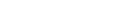Remember that parentheses stand for multiplication. Multiplying x and y may seem daunting but using the FOIL method makes things easy!

Think of the position of the x’s and the y’s above. The first position in each parenthesis consists of x’s. The last position consists of y’s.’ Using this logic, let’s apply the FOIL method: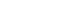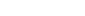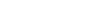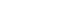Now think of the variables above as part of a large addition problem: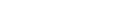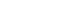## Practice FOIL-ing!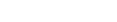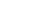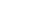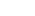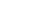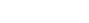You can also reverse the direction in which you do FOIL. For instance: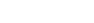First , set up the parentheses.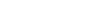The question marks are in the last position. When we multiply them together we get 4 (notice the +4 at the end of the equation). So we know that the last numbers (the question marks), when multiplied, have to equal +4.

Now note, the -4x in the middle of the equation. The outer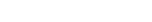Which two numbers, when multipliedf equal +4, and when added = -4. You may have to play around with the factors a little, but since the only factors of 4 are 2, 2 and 4, 1, this problem isn’t too tough. 4 and 1 would add up to 5. Therefore, 2 and 2 are the answers, and we can replace the question marks with 2’s, giving us: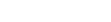## Practice Question

Which of the following is a root of the equation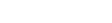1. -4
2. 2
3. 6
4. 8
5. 12You can also watch the video explanation here: https://sat.magoosh.com/questions/117

## Takeaway

Using FOIL takes a little bit of practice, but if you want to do well on the SAT you should definitely understand how it works.

## Author

•Chris Lele is the Principal Curriculum Manager (and vocabulary wizard) at Magoosh. Chris graduated from UCLA with a BA in Psychology and has 20 years of experience in the test prep industry. He's been quoted as a subject expert in many publications, including US News, GMAC, and Business Because. In his time at Magoosh, Chris has taught countless students how to tackle the GRE, GMAT, SAT, ACT, MCAT (CARS), and LSAT exams with confidence. Some of his students have even gone on to get near-perfect scores. You can find Chris on YouTube, LinkedIn, Twitter and Facebook!